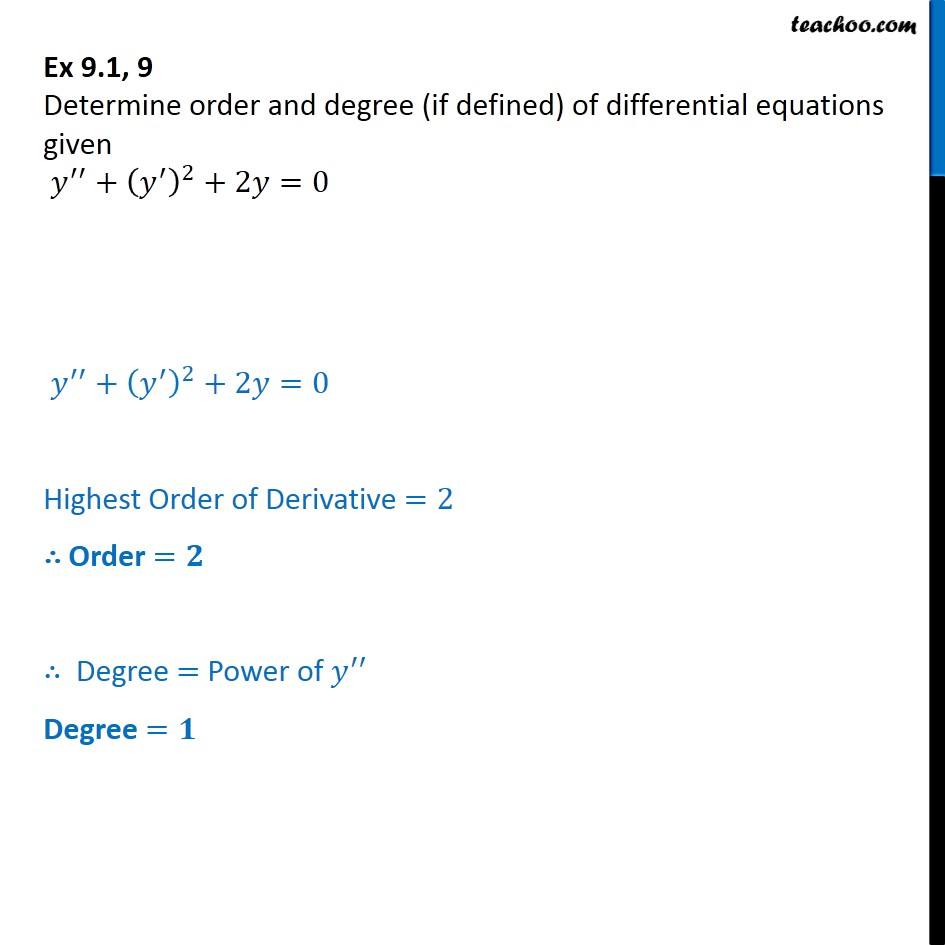Order and Degree

Chapter 9 Class 12 Differential Equations
Concept wiseIntroducing your new favourite teacher - Teachoo Black, at only ₹83 per month

### Transcript

Ex 9.1, 9 Determine order and degree (if defined) of differential equations given y′′ + ( y')′ + 2𝑦 = 0 y′′ + ( y')′ + 2𝑦 = 0 Highest Order of Derivative =2 ∴ Order =𝟐 ∴ Degree = Power of y" Degree =𝟏